# Biomedical Image Segmentation - U-Net

##### Works with very few training images and yields more precise segmentation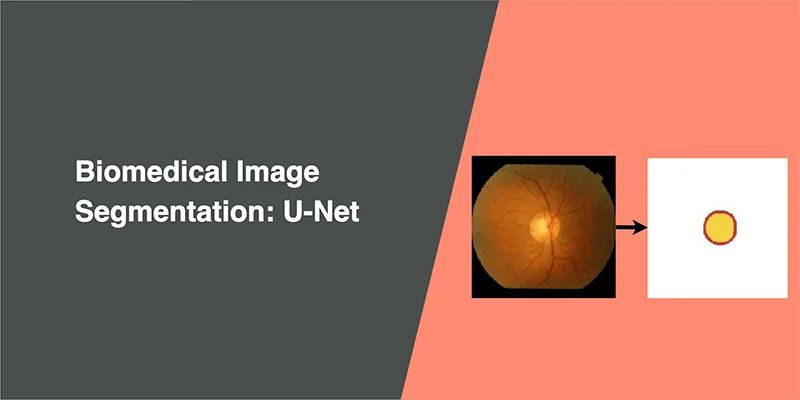# Image Segmentation

Suppose we want to know where an object is located in the image and the shape of that object. We have to assign a label to every pixel in the image, such that pixels with the same label belongs to that object. Unlike object detection models, image segmentation models can provide the exact outline of the object within an image.

## Differences between Image Classification, Object Detection and Image Segmentation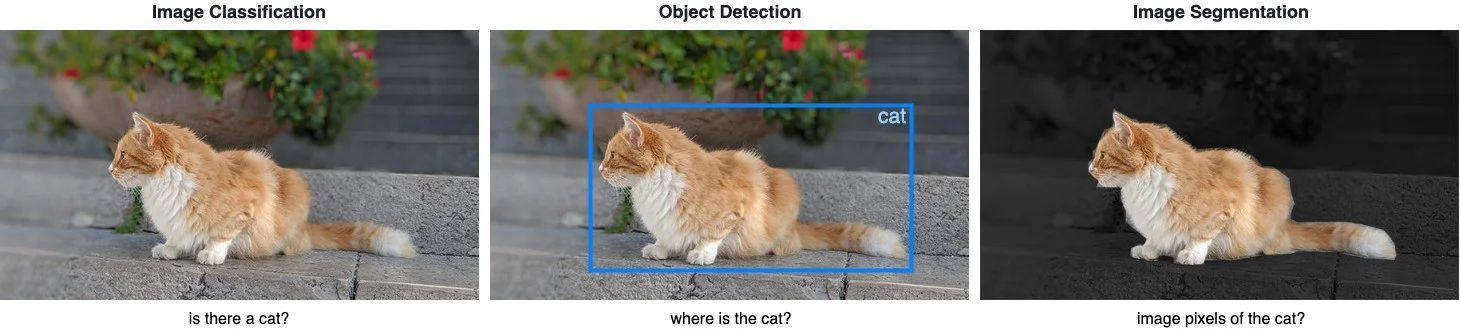Image Classification helps us to classify what is contained in an image. The goal is to answer “is there a cat in this image?”, by predicting either yes or no.

Object Detection specifies the location of objects in the image. The goal is to identify “where is the cat in this image?”, by drawing a bounding box around the object of interest.

Image Segmentation creates a pixel-wise mask of each object in the images. The goal is to identify the location and shapes of different objects in the image by classifying every pixel in the desired labels.

# U-Net

In this article, we explore U-Net, by Olaf Ronneberger, Philipp Fischer, and Thomas Brox. This paper is published in 2015 MICCAI and has over 9000 citations in Nov 2019.

U-Net is used in many image segmentation task for biomedical images, although it also works for segmentation of natural images. U-Net has outperformed prior best method by Ciresan et al., which won the ISBI 2012 EM (electron microscopy images) Segmentation Challenge.

Requires fewer training samples

Successful training of deep learning models requires thousands of annotated training samples, but acquiring annotated medical images are expansive. U-Net can be trained end-to-end with fewer training samples.

Precise segmentation

Precise segmentation mask may not be critical in natural images, but marginal segmentation errors in medical images caused the results to be unreliable in clinical settings. U-Net can yield more precise segmentation despite fewer trainer samples.

As mentioned above, Ciresan et al. worked on a neural network to segment neuronal membranes for segmentation of electron microscopy images. The network uses a sliding-window to predict the class label of each pixel by providing a local region (patch) around that pixel as input.

Limitation of related work:

• it is quite slow due to sliding window, scanning every patch and a lot of redundancy due to overlapping
• unable to determine the size of the sliding window which affects the trade-off between localization accuracy and the use of context

## Architecture

U-Net has elegant architecture, the expansive path is more or less symmetric to the contracting path, and yields a u-shaped architecture.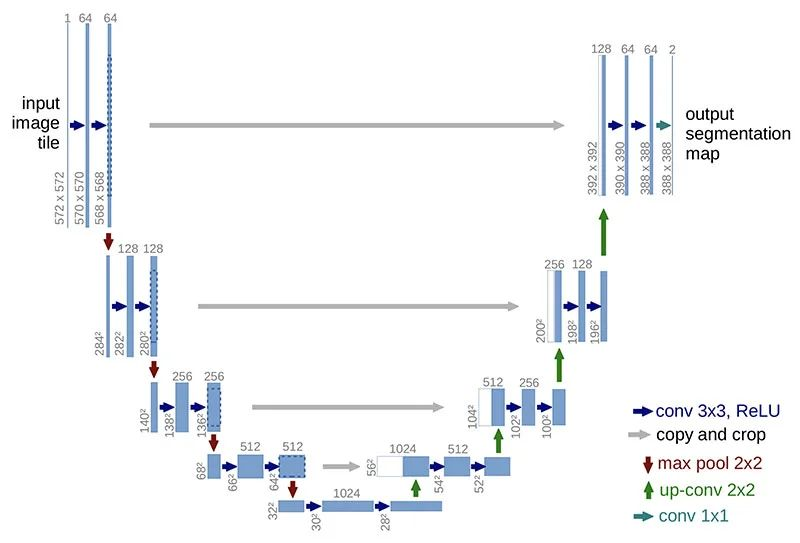Contraction path (downsampling)

Look like a typical CNN architecture, by consecutive stacking two 3x3 convolutions (blue arrow) followed by a 2x2 max pooling (red arrow) for downsampling. At each downsampling step, the number of channels is doubled.

Expansion path (up-convolution)

A 2x2 up-convolution (green arrow) for upsampling and two 3x3 convolutions (blue arrow). At each upsampling step, the number of channels is halved.

After each 2x2 up-convolution, a concatenation of feature maps with correspondingly layer from the contracting path (grey arrows), to provide localization information from contraction path to expansion path, due to the loss of border pixels in every convolution.

Final layer

A 1x1 convolution to map the feature map to the desired number of classes.

# My experiment on U-Net

I will be using the Drishti-GS Dataset, which is different from what Ronneberger et al. have used in their paper. This dataset contains 101 retina images, and annotated mask of the optical disc and optical cup, for detecting Glaucoma, one of the major cause of blindness in the world. 50 images will be used for training, and 51 for validation.

## Metrics

We need a set of metrics to compare different models, here we have Binary cross-entropy, Dice coefficient and Intersection over Union.

Binary cross-entropy

A common metric and loss function for binary classification for measuring the probability of misclassification.

We will be using binary_cross_entropy_with_logits from PyTorch. Used together with the Dice coefficient as the loss function for training the model.

Dice coefficient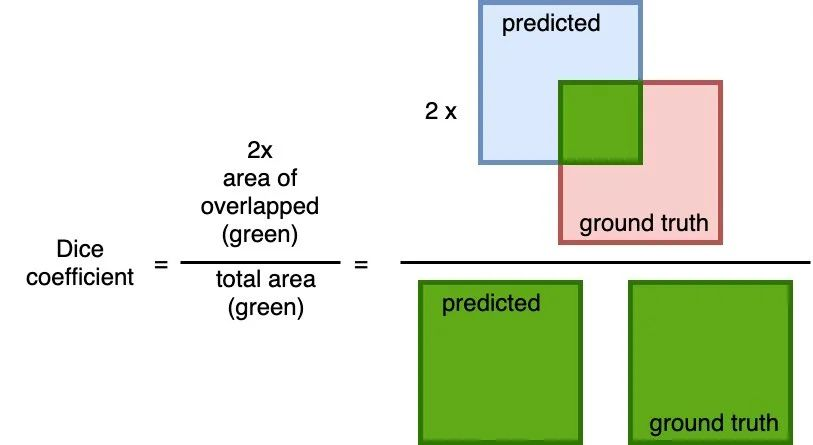A common metric measure of overlap between the predicted and the ground truth. The calculation is 2 * the area of overlap (between the predicted and the ground truth) divided by the total area (of both predict and ground truth combined).

This metric ranges between 0 and 1 where a 1 denotes perfect and complete overlap.

I will be using this metric together with the Binary cross-entropy as the loss function for training the model.

Intersection over Union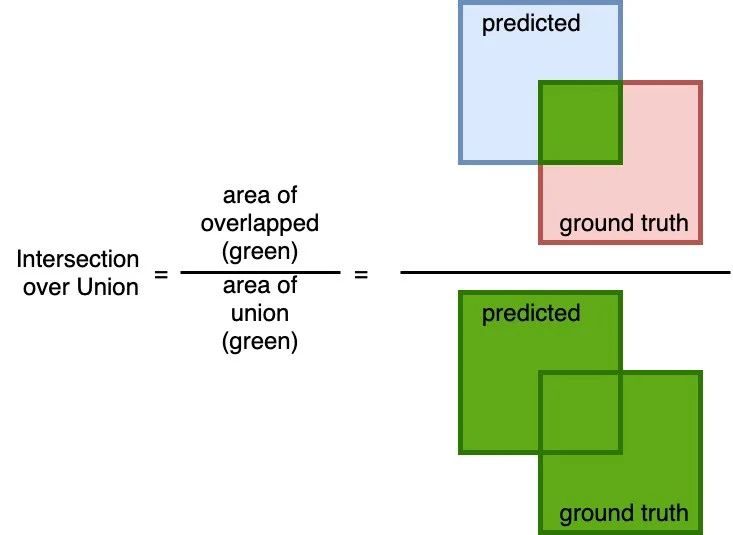A simple (yet effective!) metric to calculate how accurate the predicted mask is with the ground truth mask. The calculation to compute the area of overlap (between the predicted and the ground truth) and divide by the area of the union (of predicted and ground truth).

Similar to the Dice coefficient, this metric range from 0 to 1 where 0 signifying no overlap whereas 1 signifying perfectly overlapping between predicted and the ground truth.

## Training and results

To optimize this model as well as subsequent U-Net implementation for comparison, training over 50 epochs, with Adam optimizer with a learning rate of 1e-4, and Step LR with 0.1 decayed (gamma) for every 10 epochs. The loss function is a combination of Binary cross-entropy and Dice coefficient.

The model completed training in 11m 33s, each epoch took about 14 seconds. A total of 34,527,106 trainable parameters.The epoch with the best performance is epoch #36 (out of 50).

• Binary cross-entropy: 0.3319
• Dice coefficient: 0.8367
• Intersection over Union: 0.8421

Test the model with a few unseen samples, to predict optical disc (red) and optical cup (yellow).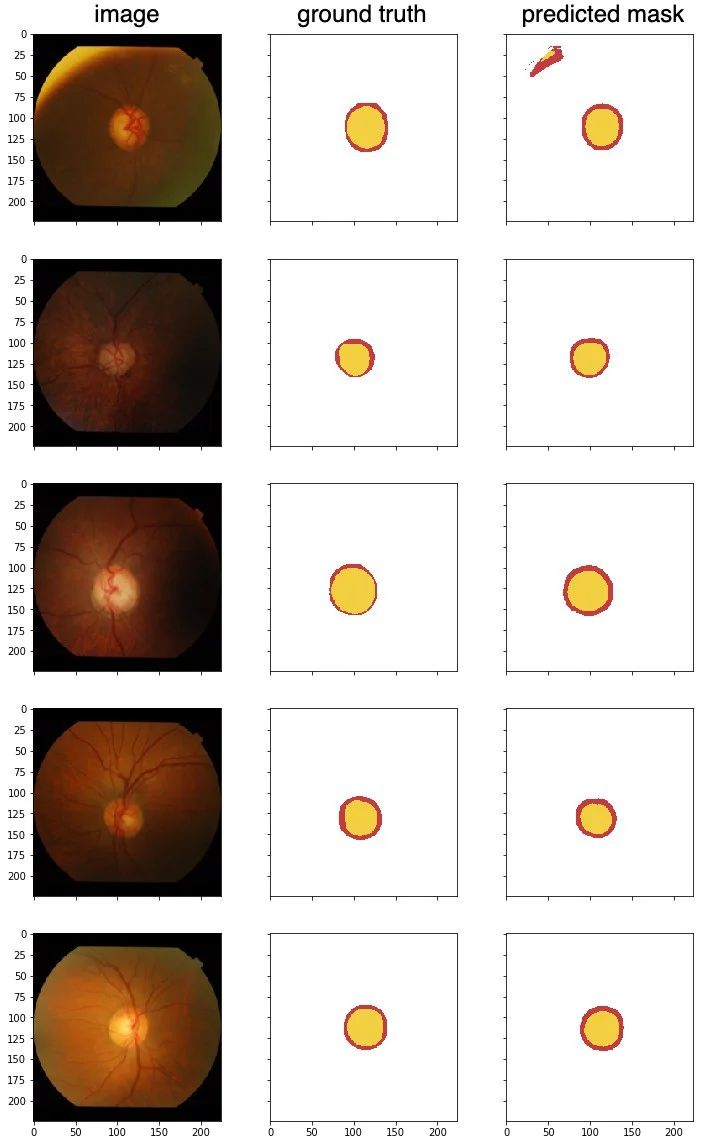From these test samples, the results are pretty good. I chose the first image because it has an interesting edge along the top left, there is a misclassification there. The second image is a little dark, but there are no issues getting the segments.

# Conclusion

U-Net architecture is great for biomedical image segmentation, achieves very good performance despite using only using 50 images to train and has a very reasonable training time.

Here is the PyTorch code of U-Net: